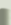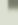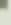# C o m p l e x      A n a l y s i s

homeAll material is also available in Moodle.

## OVERVIEW

This page will be used to make announcements and provide copies of handouts, lecture notes, problem sheets and their solutions for this course. Files will be supplied in pdf format.

I welcome feedback in the form of constructive comments or criticism. Just send an email to or talk to me after the lectures.

Information handout

Mathematical questions (e.g., received by email) on statements in the lecture are answered in the FAQ-pdf, see Section "Lecture Notes".

Exam papers of the previous years can be found on the Library and Learning Centre Exam Papers Database (type in "MA30056").

 new Here is this year's final exam and my feedback on it and your comments in the course evaluation. I still welcome any fair comment (at E-Mail-Address secured by JavaScript. Please enable JavaScript. ). new

### LECTURE NOTES

Can be found here (version: 27 Apr).
If you print the lecture notes you might want to exclude pp. 26-33 & pp. 61-62 & pp. 82-83.

Note: Lecture notes are not a textbook! There might be misprints as well as mathematical errors (please do notify me, preferrably by email, if you spot an error, even if it is only a minor misprint).

On the exercises in the lecture notes: see bottom of page.

FAQs on statements in the lecture are answered here (version: 19 May).

### EXERCISE SHEETS

Exercise sheet 10 with solutions (questions only)

Exercise sheet 9 with solutions (questions only)

Exercise sheet 8 with solutions (questions only)

Exercise sheet 7 with solutions (questions only)

Exercise sheet 6 with solutions (questions only)

Exercise sheet 5 with solutions (questions only)

Exercise sheet 4 with solutions (questions only)

Exercise sheet 3 with solutions (questions only)

Exercise sheet 2 with solutions (questions only)

Exercise sheet 1 with solutions (questions only)

### SELF-ASSESSMENT SHEETS

Self-assessment sheet 10

Self-assessment sheet 9

Self-assessment sheet 8

Self-assessment sheet 7

Self-assessment sheet 6

Self-assessment sheet 5

Self-assessment sheet 4

Self-assessment sheet 3

Self-assessment sheet 2

Self-assessment sheet 1

### REVISION MATERIAL

Checklist & Previous Exam Questions I

Checklist & Previous Exam Questions II

Overview of this unit (slides used in revision session)

Some mistakes in Questions 1 & 2 on Exercise sheet 7

Wikipedia and mathworld on Apéry's constant ζ(3).

More proofs of ζ(2)=∑ 1⁄n² = π²⁄6 can be found here.

Handout "Evaluating ζ(2)=∑ 1⁄n²"

Handout "Evaluating ∫dx⁄(1+x4)"

Handout "Singularities"

Here is a Java applet that let's you explore some Laurent series (author is again Terence Tao).

An animation on what is happening in Question 3 on Exercise sheet 8.

Here is a Java applet that let's you explore some multifunctions. By the way, the author is Terence Tao.

Handout showing the principal values of the logarithm and the square root

Here is a physics YouTube video demonstrating what you can do with a laminar fluid

"Mathlets" that demonstrate that f(x)=x sin(1/x) is not differentiable at 0 while g(x)=x2 sin(1/x) is, can be found here.

Here is a link to an article that uses level curves to study Riemann's zeta function (written for students)

Here is a Java Applet that lets you draw the image of vertical/horizontal lines (and circles) under some holomorphic functions

Handout "Cauchy-Riemann revisited"

Here is a really nice YouTube video explaining Möbius transformations

Handout "Derivative of f:ℝ2→ℝ2"

Handout "Stereographic Projection"

### DIARY

27.4.: characterization of singularities; meromorphic functions; residues; Residue Theorem; evaluation of real integrals; calculation of ζ(2)=∑1⁄n² = π²⁄6
20.4.: Laurent series; annulus of convergence; holomorphicity of functions defined by Laurent series; Laurent's Theorem; isolated singularities (removable singularity, pole, essential singularity)
16.3.: holomorphicity of (locally) uniform limits of holomorphic functions; power series and the Weiserstrass M-test; holomorphicity of functions defined by power series; the Cauchy-Taylor Theorem; analytic functions; the Identity Theorems for power series and for holomorphic functions; the Maximum Modulus Theorem
9.3.: Cauchy's inequalities; Lioville's Theorem; Gauss' Fundamental Theorem of Algebra; local Maximum Modulus Theorem; evaluating integrals using Cauchy's Formulae; applications in physics; logarithms, powers and multifunctions; (local) uniform convergence
2.3.: star-domains; homotopy version of Cauchy's Theorem; Cauchy's Formulae; Morera's Theorem
23.2.: fundamental theorem of calculus for path integrals; criterium for FTC Cauchy's Theorem; Cauchy's Theorem in simply connected domains (when f' is continuous); Cauchy-Goursat Theorem
16.2.: complex exponential, cosine and sine function; path and contour integrals; ML-inequality
9.2.: real & complex differentiability; necessary and sufficient Cauchy-Riemann conditions; geometric meaning of Cauchy-Riemann equations
2.2.: paths, curves, contours and domains; continuous complex functions; (complex) differentiation
29.1.: topology of the complex numbers; stereographic projection & extended complex plane
26.1.: introduction & overview; algebra & geometry of the complex numbers

On the exercises in the lecture notes:

• Clearly, exercises that appear on the exercise sheet are examinable.
• Exercises set during the lecture (but not on the exercise sheet) are essentially of two kinds:
1. Refering to previous lectures ("... as in M11"). If you don't know how to solve them, please consult your notes of that course. I assume that you have a "working knowledge" of the corresponding statements (e.g., you know how to apply the chain rule, but you might not know all the details of its proof; so exercises refering to such proofs are not examinable).
2. Chapter I. provides auxiliaries for things we do later on. Again, we need a "working knowledge" of these, but a detailed proof in this (complex analysis!) lecture would be distracting (e.g., the proof of Theorem I.5.1 belongs to a [point set] topology course, but of course we will work in domains from now on). However, if you are interested in these proofs, then the exercise/drop-in session is a good place!
However, model solutions to these exercises will not be given (only to the ones on the exercise sheet).

© 2008 by Bernd Sing • • last update: 30.05.2009 (05/30/2009)